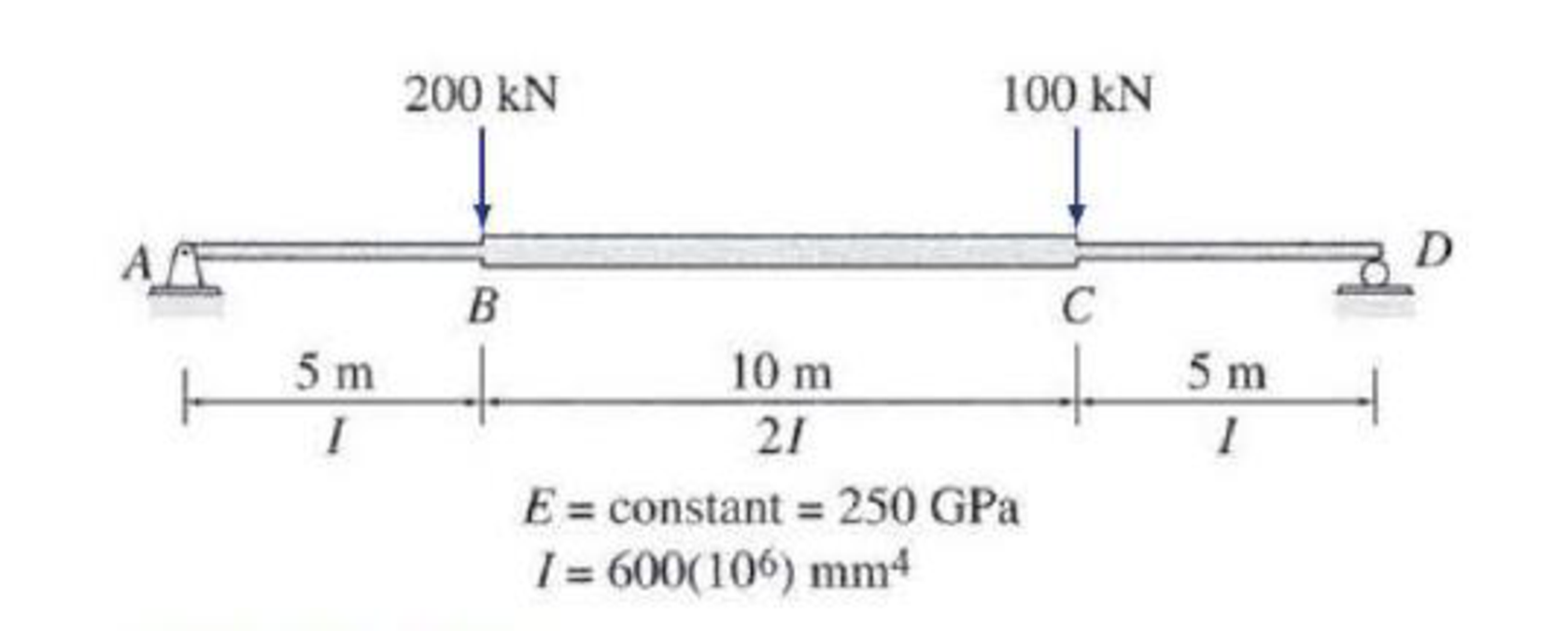# 7.59 through 7.62 Use Castigliano’s second theorem to determine the deflection at point C of the beams shown in Figs. P7.24−P7.27.

#### Solutions

Chapter
Section
Chapter 7, Problem 61P
Textbook Problem
60 views

## 7.59 through 7.62 Use Castigliano’s second theorem to determine the deflection at point C of the beams shown in Figs. P7.24−P7.27.To determine

Find the deflection at point C of the beam using Castigliano’s second theorem.

### Explanation of Solution

Given information:

The beam is given in the Figure.

The value of E is 250 GPa and I is 600×106mm4.

Apply the sign conventions for calculating reactions, forces and moments using the three equations of equilibrium as shown below.

• For summation of forces along x-direction is equal to zero (Fx=0), consider the forces acting towards right side as positive (+) and the forces acting towards left side as negative ().
• For summation of forces along y-direction is equal to zero (Fy=0), consider the upward force as positive (+) and the downward force as negative ().
• For summation of moment about a point is equal to zero (Matapoint=0), consider the clockwise moment as negative and the counter clockwise moment as positive.

Calculation:

Let apply a load P at point C in the desired direction to find the deflection.

The value of load P is 100 kN.

Sketch the beam with load P as shown in Figure 1.

Let the equation for bending moment at distance x in terms of load P be M, the derivative of M with respect to P is MP.

Find the reactions at the supports A and D:

Summation of moments about A is equal to 0.

MA=0Dy(20)P(15)200(5)=020Dy=1,000+15PDy=50+3P4

Summation of forces along y-direction is equal to 0.

+Fy=0Ay+Dy200P=0Ay+50+3P4200P=0Ay=150+P4

Find the equations for M and MP for the 3 segments of the beam as shown in Table 1.

 Segment x-coordinate M ∂M∂P Origin Limits (m) AB A 0−5 (150+P4)x 0.25x BC A 5−15 (150+P4)x−200(x−5) 0.25x DC D 0−5 (50+3P4)x 0.75x

Refer to Table 1.

The value of load P is 100 kN.

For span AB,

M=(150+1004)x=175x

For span BC,

M=(150+1004)x200(x5)=175x200x+1,000=25x+1,000

For span DC,

M=(50+3(100)4)x=125x

The expression for deflection at C using Castigliano’s second theorem (ΔC) is shown as follows:

ΔC=0L(MP)MEIdx (1)

Here, L is the length of the beam.

For span AB moment of inertia is 2I and for span BC the moment of inertia is I.

Substitute I for span AB, 2I for span BC, and I for span CD.

Rearrange Equation (1) for the limits 05, 515, and 05 as follows.

ΔC=1EI[05(MP)Mdx+12515(MP)Mdx+05(MP)Mdx]

Substitute 0

### Still sussing out bartleby?

Check out a sample textbook solution.

See a sample solution

#### The Solution to Your Study Problems

Bartleby provides explanations to thousands of textbook problems written by our experts, many with advanced degrees!

Get Started

Find more solutions based on key concepts
Give examples of the units of length in SI and U.S. Customary systems.

Engineering Fundamentals: An Introduction to Engineering (MindTap Course List)

Compute the nominal flexural strength of the composite beam in Problem 9.1-3. Use Fy=50 ksi.

Steel Design (Activate Learning with these NEW titles from Engineering!)

Precision Machining Technology (MindTap Course List)

Faulty Update Your computer is set to install updates for the operating system, programs, and apps automaticall...

Enhanced Discovering Computers 2017 (Shelly Cashman Series) (MindTap Course List)

If your motherboard supports ECC DDR3 memory, can you substitute non-ECC DDR3 memory?

A+ Guide to Hardware (Standalone Book) (MindTap Course List)

What is the maximum width of the cover pass? Why?

Welding: Principles and Applications (MindTap Course List)